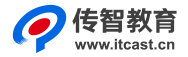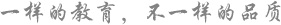## PHP产生不重复随机数的5个方法总结

\$numbers = range (1,50);

//shuffle 将数组顺序随即打乱

shuffle (\$numbers);

//array_slice 取该数组中的某一段

\$num=6;

\$result = array_slice(\$numbers,0,\$num);

print_r(\$result);

?>

\$numbers = range (1,20);

//播下随机数发生器种子，可有可无，测试后对结果没有影响

srand ((float)microtime()*1000000);

shuffle (\$numbers);

//跳过list第一个值(保存的是索引)

while (list(, \$number) = each (\$numbers)) {

echo "\$number ";

}

?>

function NoRand(\$begin=0,\$end=20,\$limit=5){

\$rand_array=range(\$begin,\$end);

shuffle(\$rand_array);//调用现成的数组随机排列函数

return array_slice(\$rand_array,0,\$limit);//截取前\$limit个

}

print_r(NoRand());

?>

\$tmp=array();

while(count(\$tmp)<5){

\$tmp[]=mt_rand(1,20);

\$tmp=array_unique(\$tmp);

}

print_r(\$tmp);

?>

\$tmp = range(1,30);

print_r(array_rand(\$tmp,10));

?>